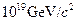Chapter 34, Problem 13PE

Chapter
Section
Textbook Problem

The average particle energy needed to observe uni?cation of forces is estimated to be 1019 GeV. (a) What is the rest mass in kilograms of a particle that has a rest mass of(b) How many times the mass of a hydrogen atom is this?

To determine

(a)

The rest mass in kilograms.

Explanation

Given data:

Velocity of light is c=3×108m/s.

Formula used:

The given formula for rest mass is,

M=1019GeVc2   .........(1)

Calculation:

Substitute the given value in equation (1).

M= 10 19×( 1.6× 10 19 C)( 10</

To determine

(b)

The rest mass of particle as a multiple of mass of hydrogen atom.

Still sussing out bartleby?

Check out a sample textbook solution.

See a sample solution

The Solution to Your Study Problems

Bartleby provides explanations to thousands of textbook problems written by our experts, many with advanced degrees!

Get Started